Home Page

Normalizing Flows have been all the rage lately and with all new breeds of generative models come new avenues for their application. Recently, there’s been a burgeoning interest in bringing in tools from differential geometry in order to do effective Deep Learning on non-Euclidean manifolds. For the purposes of this blog post, I’ll focus on one recent extension of Normalizing Flows to hyperbolic spaces based on my recent ICML 2020 paper titled “Latent Variable Modeling with Hyperbolic Normalizing Flows” While a deep knowledge of Riemannian geometry is not needed it definitely helps in understandings a lot of the intuition and technical details. In this blog post I’ll try to distill the key concepts but to get the most out of the material I highly recommend the following blog posts as well to get a feel for Hyperbolic Geometry:

# Motivation

To motivate why we might want to care about geometry when doing generative modeling we can simply look at different domains that have seen remarkable breakthroughs.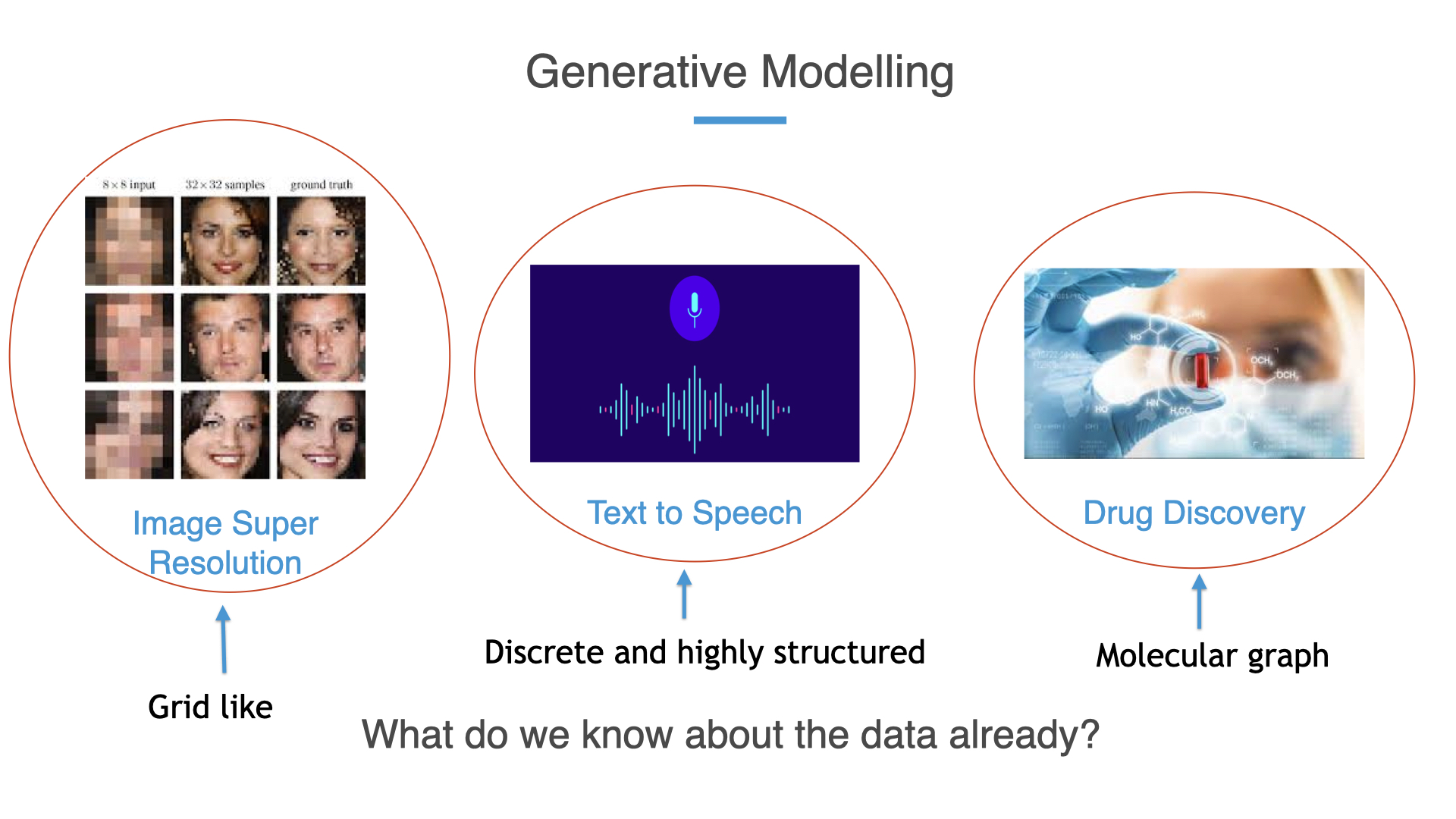But in all these different domains, we can ask the following question: “What do we know about the data already?”. For instance, images are known to be very grid-like, text is very discrete, and structured (and an arbitrary assortment of words don’t usually make a sentence) and even molecules can be represented as graphs. Data in these domains have vastly different geometry, so the natural question is shouldn’t any generative model being trained also be privy this geometry? Sometimes as practitioners we can neglect what is already available to us, and neglecting known geometry in the data unnecessarily makes the learning problem significantly harder.

# Hierarchical structure and Hyperbolic Geometry

Turning to a concrete use case, what if we know apriori that our data is tree-like or has a rich hierarchical structure? What is the right geometry here? Well to gain intuition we can first see what can go wrong when we naively try to embed a tree in Euclidean space.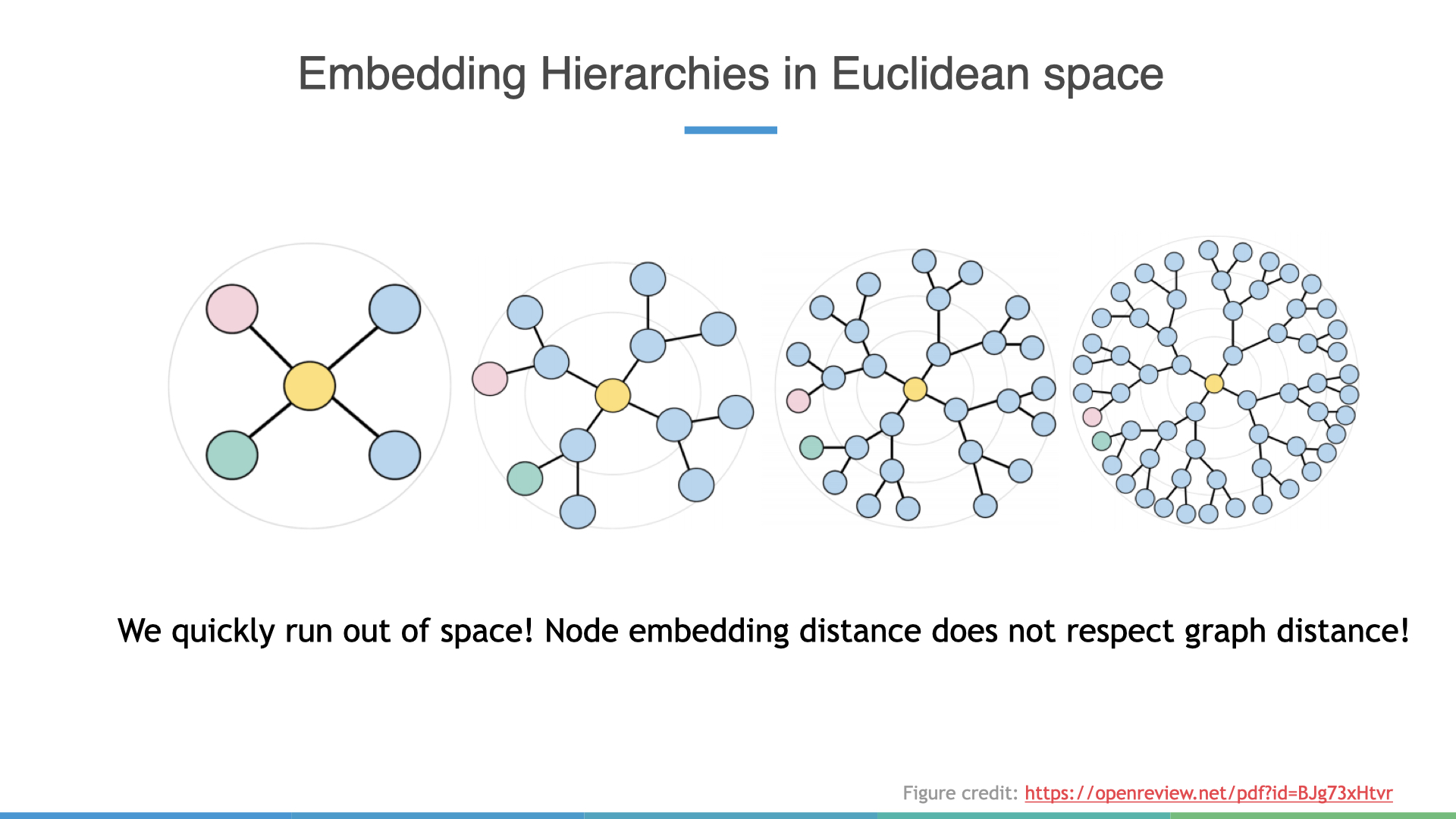In this construction, we evenly place all leaves around the circumference of an imaginary circle with radius $$r$$. Observe how the Euclidean distance between the pink and green nodes decreases as we increase the depth of the tree but the graph distance —i.e. shortest path between these nodes, actually increases! Clearly, Euclidean space is not respecting this notion of graph distance well. This happens to be a fundamental limitation of Euclidean spaces, and the hand-wavy answer to this phenomenon is because Euclidean spaces are not growing fast enough to accommodate the exponential growth of nodes. I love this figure (taken from fig) because it succinctly encapsulates the problem in embedding hierarchies in Euclidean.

So lets see how hyperbolic spaces alleviates this problem: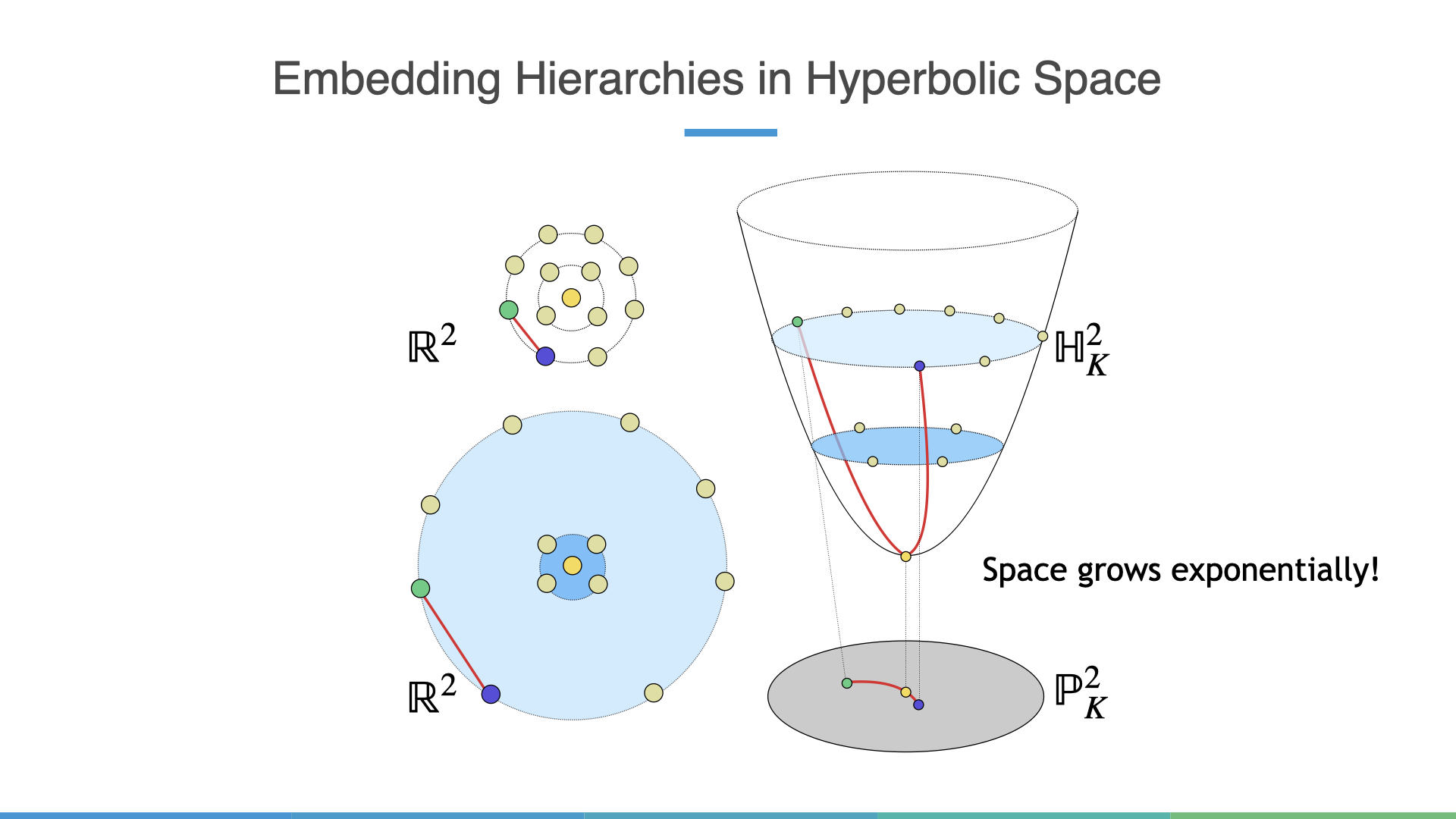One important fact to realize is that because hyperbolic spaces are manifolds of constant negative curvature many of the geometric intuitions from Euclidean spaces go out the window. For instance, the shortest path between two points is now a curved path (geodesic)! It just so happens the shortest path between two nodes in a tree must go through a common parent, in this case, this is the root node, which matches our intuition of graph distances.

# Latent Variable Modeling and Normalizing Flows

Now consider the problem of latent variable modeling in the canonical VAE setting. To train these models we often optimize for the Evidence Lower Bound (ELBO) objective:

\begin{align*} \mathbb{E}_{q_i(z)}\Big[\log \frac{p(x|z)p(z)}{q_i(z)}\Big] = \mathbb{E}_{q_i(z)}[\log p(x_i|z)] - D_{KL}(q_i || p) \end{align*}

But what have we already implicitly assumed in this formulation? All densities are taken to be Euclidean densities even though the data can be highly non-Euclidean! Even learning more flexible approximate posteriors through Normalizing Flows cannot always overcome this fundamental geometric limitation. What we really need is to learn densities on manifolds and in the particular case of this blog post hyperbolic manifolds.

While there is an abundance of material on understanding normalizing flows they can really be understood in terms of 3 key desiderata:

• Each function $$f_i$$￼ must be invertible.
• We must be able to efficiently sample from the final distribution $$z_j = f_j \circ f_{j-1} \circ \dots \circ f_1(z_0)$$
• We must be able to efficiently compute the associated change in volume
\begin{align*} \log p(z_j) = \log p(z_0) - \sum_{i=1}^k\log det\Big|\frac{\partial f_j}{\partial z_{j-1}} \Big| \end{align*}

Thus normalizing flows compose several invertible simple functions to construct an arbitrarily complex function which allows one to sample from a simple base distribution but yields a sample from a significantly more complex distribution. All of this is hinged on the change in volume formula for probability distributions which can be extremely efficient to compute given certain types of $$f_i$$’s. A concrete instantiation of this is the now famous RealNVP or Affine coupling flow that basically partitions an input vector into two sets. The first set undergoes an identity map while the second set is pushed through a scale ($$s$$) and translation ($$t$$) transformation conditioned on the first.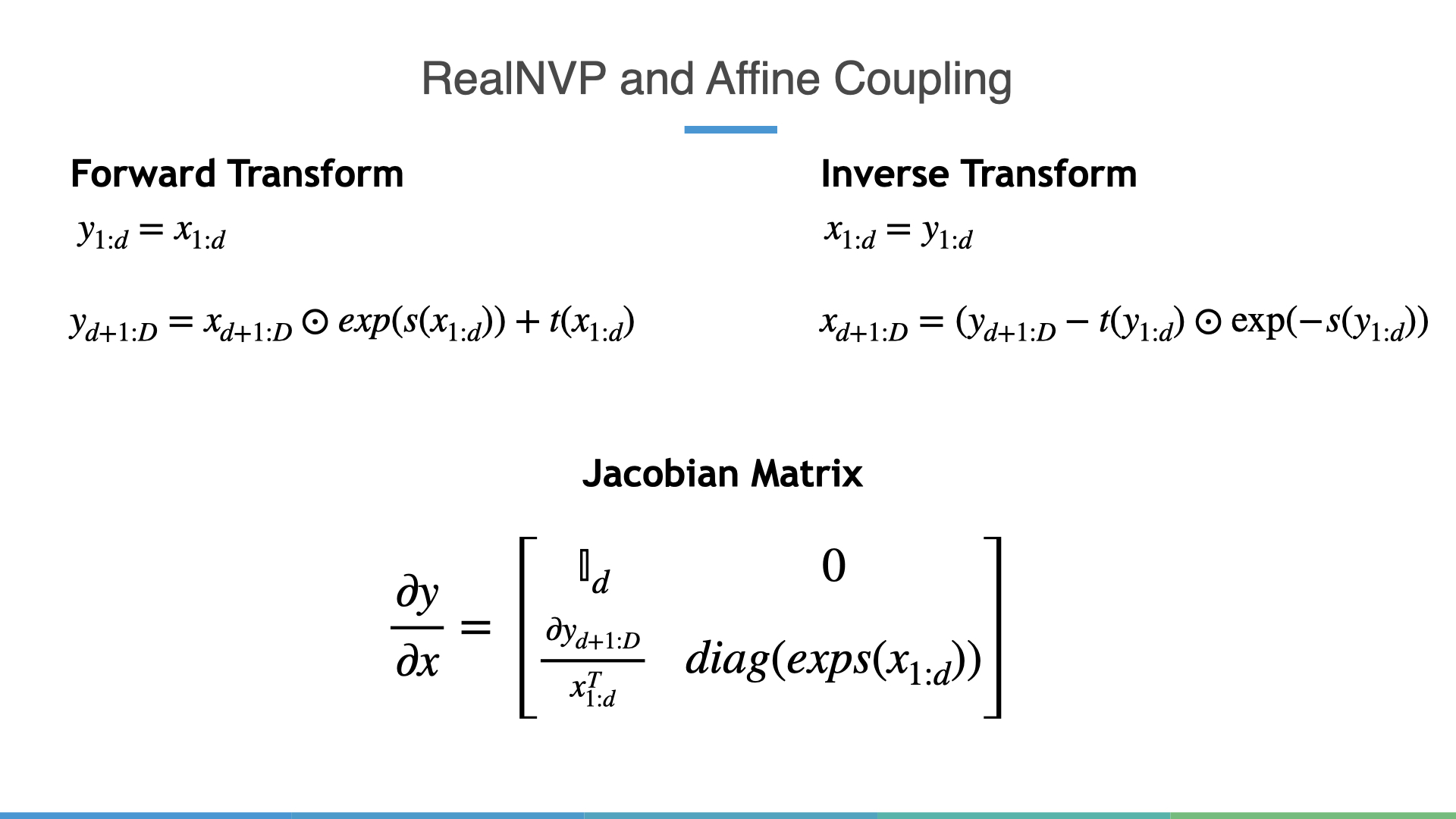Notice how the Jacobian Matrix has a nice lower triangular form, which allows for an efficient calculation of the change in volume.

# Quick Primer on Lorentz Model of hyperbolic geometry

Hyperbolic geometry in itself is a vast and rich topic that avid scholars can pour years of their life into. For the purposes of this blog post, I’ll outline the spark notes version and refer the interested reader to the Appendix of the paper.

There are many equivalent models of hyperbolic geometry, but the Lorentz model offers the simplest explicit formulas. At its core the hyperboloid manifold is equipped with the Lorentz Inner Product:

\begin{align*} \langle \textbf{x}, \textbf{y} \rangle_{\mathcal{L}} := -x_0y_0 + x_1y_1 + \dots + x_ny_n, \end{align*}

With this inner product (sometimes called metric) we can now define familiar notions of distances and angles in hyperbolic space. Loosely speaking when a smooth manifold equipped this metric is also a Riemannian manifold. Formally, the hyperboloid with curvature constant $$K$$ is defined as:

\begin{align*} \mathbb{H}^{n}_K := \{x \in \mathbb{R}^{n+1}: \langle \textbf{x}, \textbf{x} \rangle_{\mathcal{L}} = 1/K, \ x_0 > 0, \ K<0 \} \end{align*}

## Tangent Spaces and Parallel Transport

In laymans terms the tangent space at a point $$\mu$$ is a Euclidean space spanned by all tangent vectors to the manifold at $$\mu$$. In pictures this is: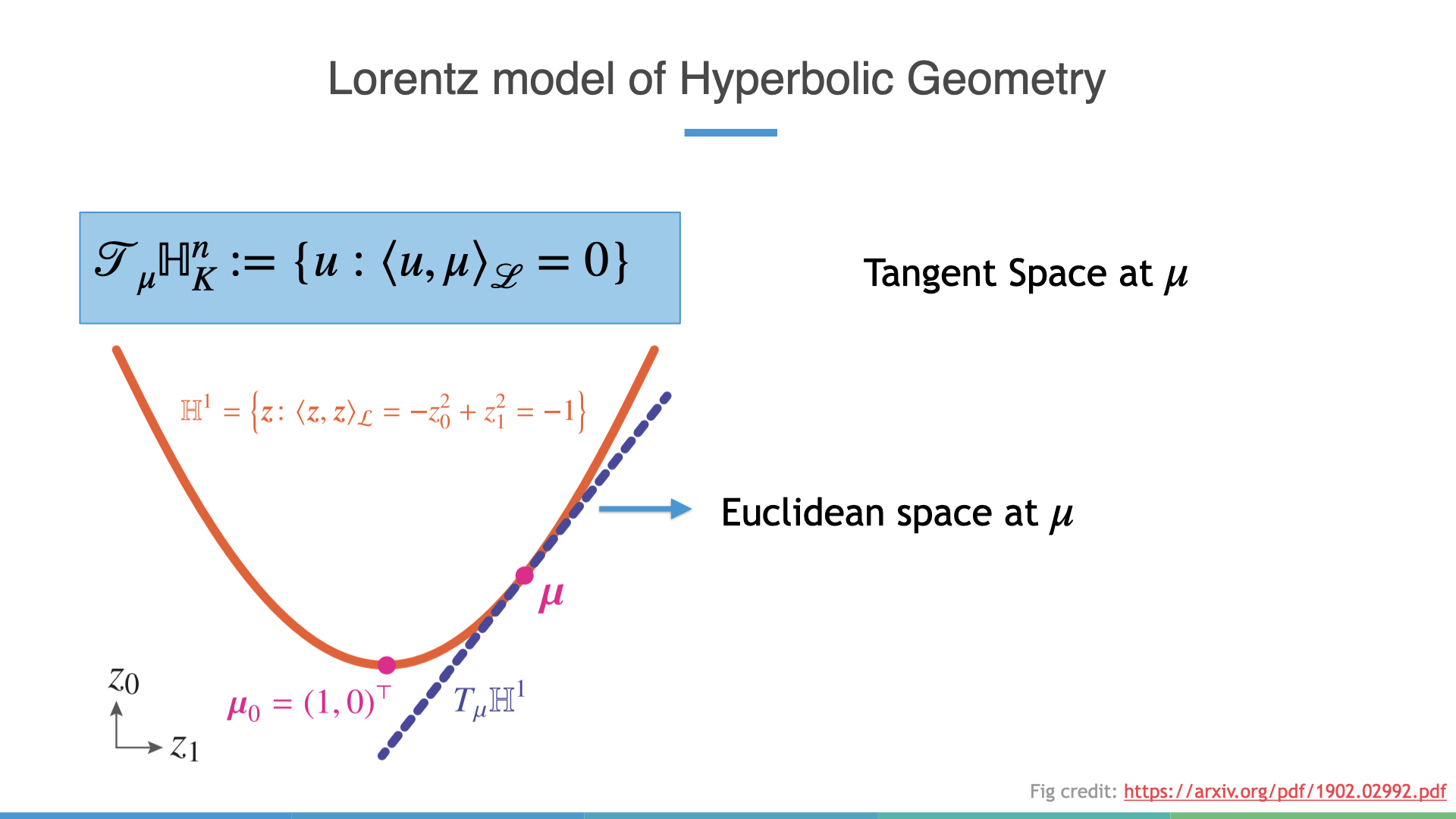We may also move vectors between tangent spaces. The operation that does this but “preserves” the metric is known as parallel transport. We’ll see later on that parallel transport doesn’t induce any change in volume.

## Exponential and Logarithmic Maps

We can also move vectors from the tangent space at a point to the manifold and vice-versa. Mapping a point from the tangent space to the manifold is known as the exponential map, while the inverse operation is called the logarithmic map. It’s important to note that both maps may not have closed-form solutions for arbitrary Riemannian manifolds, but luckily do have nice closed forms in the Lorentz model.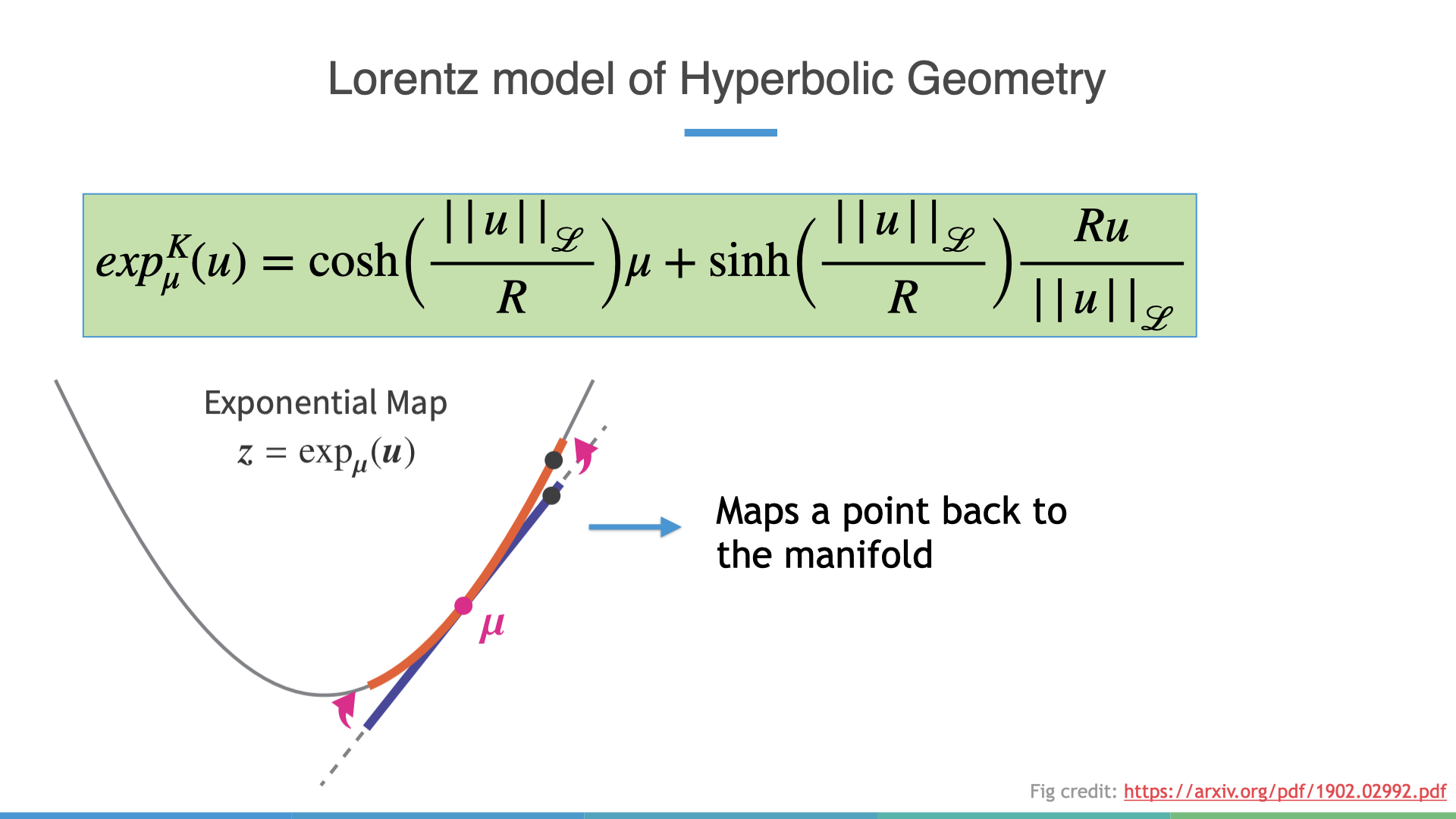## Distributions on Hyperbolic Spaces

Now finally we can talk about one concrete way to define distributions on Hyperbolic spaces. This construction follows from the following papers nagano_et_al_2019. I think this figure does a pretty good job at explaining the idea: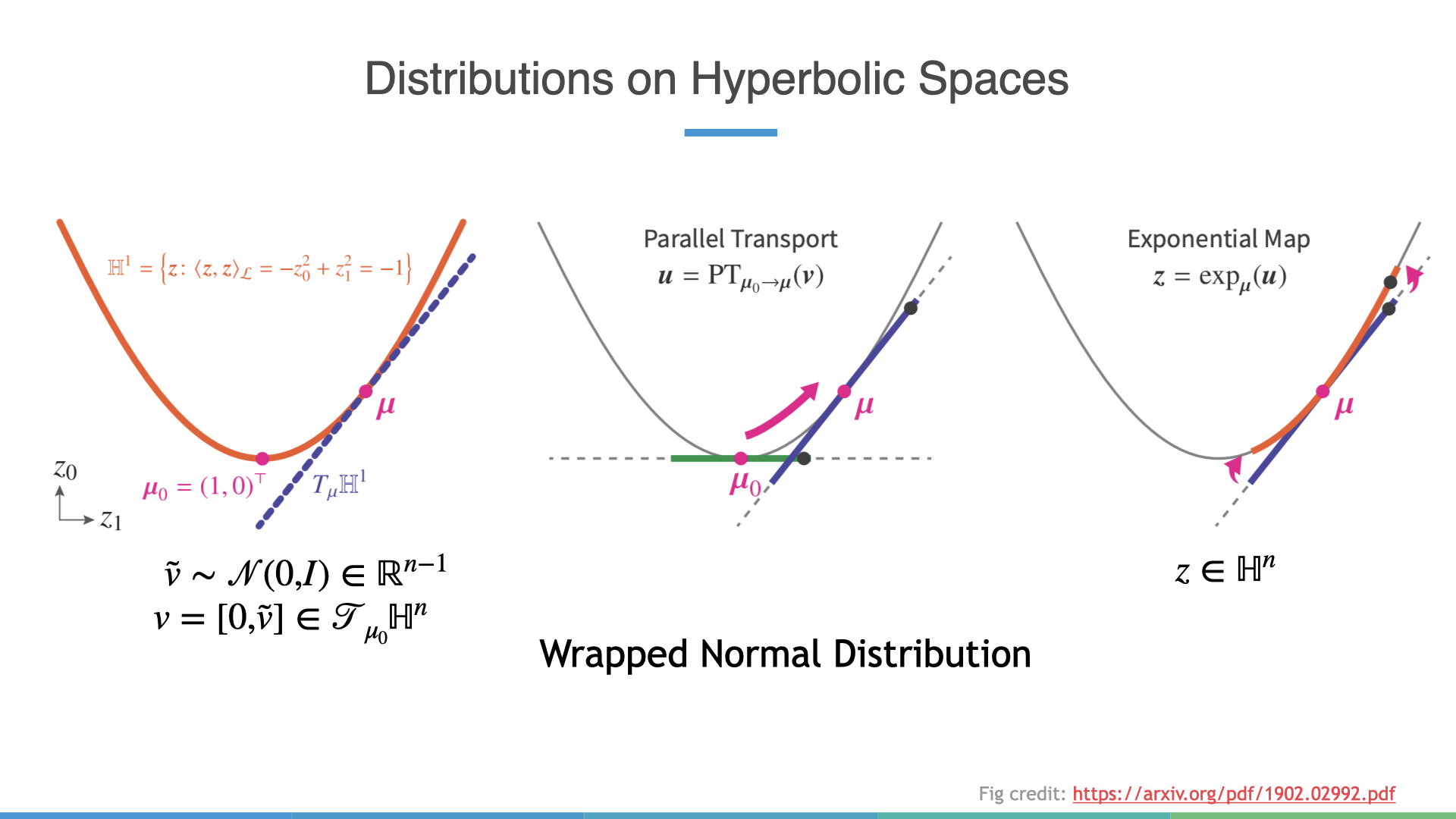Essentially, we sample from a standard normal distribution with one less dimension. Afterwhich, we prepend a $$0$$ element to the sample $$\tilde{v}$$ which allows us to reinterpret this as a sampled vector residing at the tangent space the origin. We can then use parallel transport to move this vector to a pre-learned “mean” parameter’s tangent space ($$\mu$$), before finally applying the exponential map to get a vector on the hyperboloid. In the literature, this is known as a Wrapped Normal Distribution.

# Normalizing Flows on Hyperbolic Spaces: Tangent Coupling

We’re finally ready to define our first normalizing flow on hyperbolic spaces! Let’s quickly recap the key challenges. First, $$\mathbb{H}^n_K$$ doesn’t have vector space structure, making it hard to apply conventional deep learning techniques. However, the tangent space at the origin does so we can liberally make use of it. Second, we want a final sample to reside on the actual manifold. Like the Wrapped Normal, we simply use exponential and logarithmic maps to move between tangent spaces and the actual manifold.

So given these ideas we can now define a new Normalizing Flow layer termed: “Tangent Coupling”.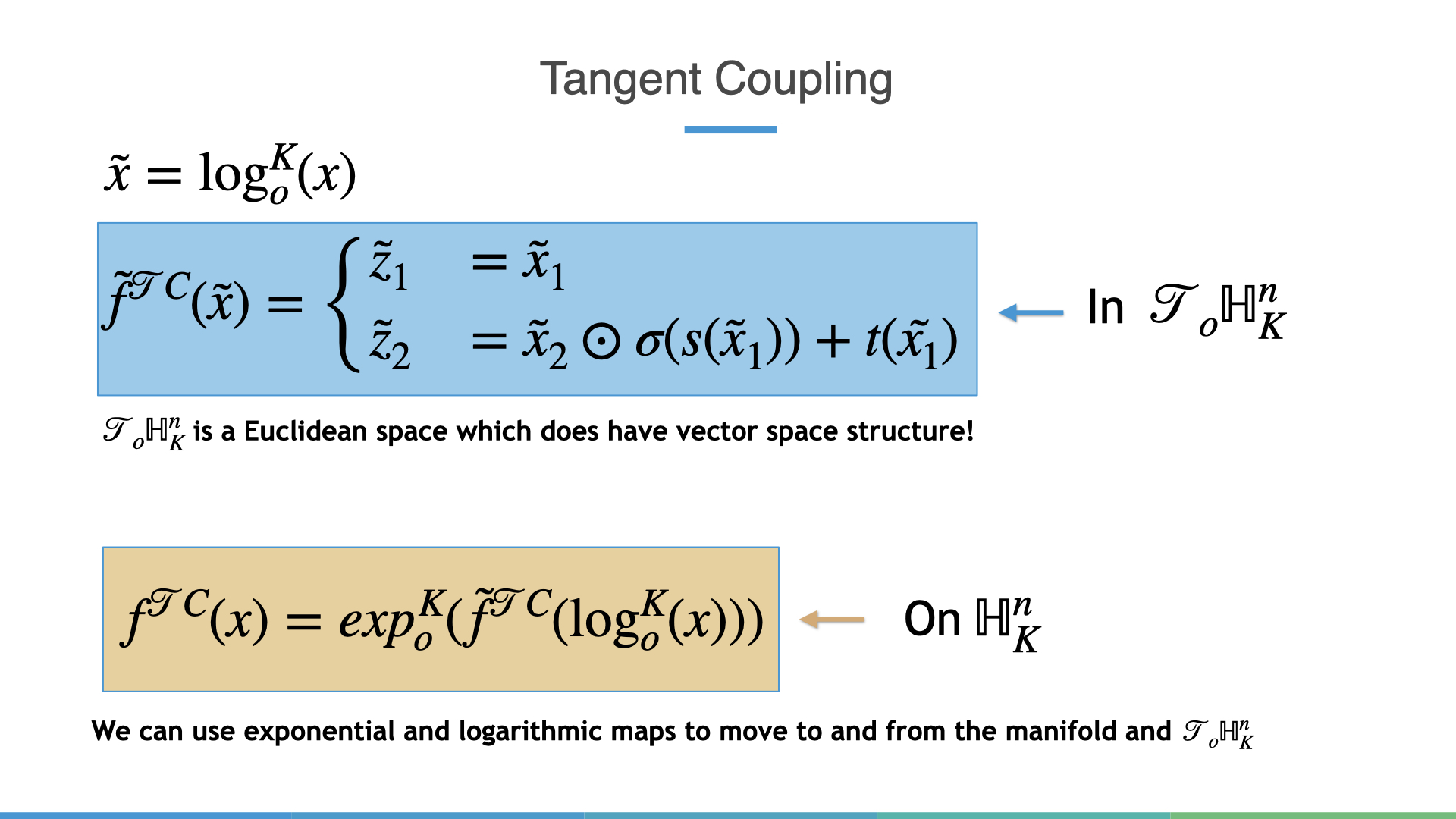One might think that the change in volume due to a single Tangent Coupling layer might be expensive but as it turns out, just like regular Affine coupling it is $$\mathcal{O}(n)$$.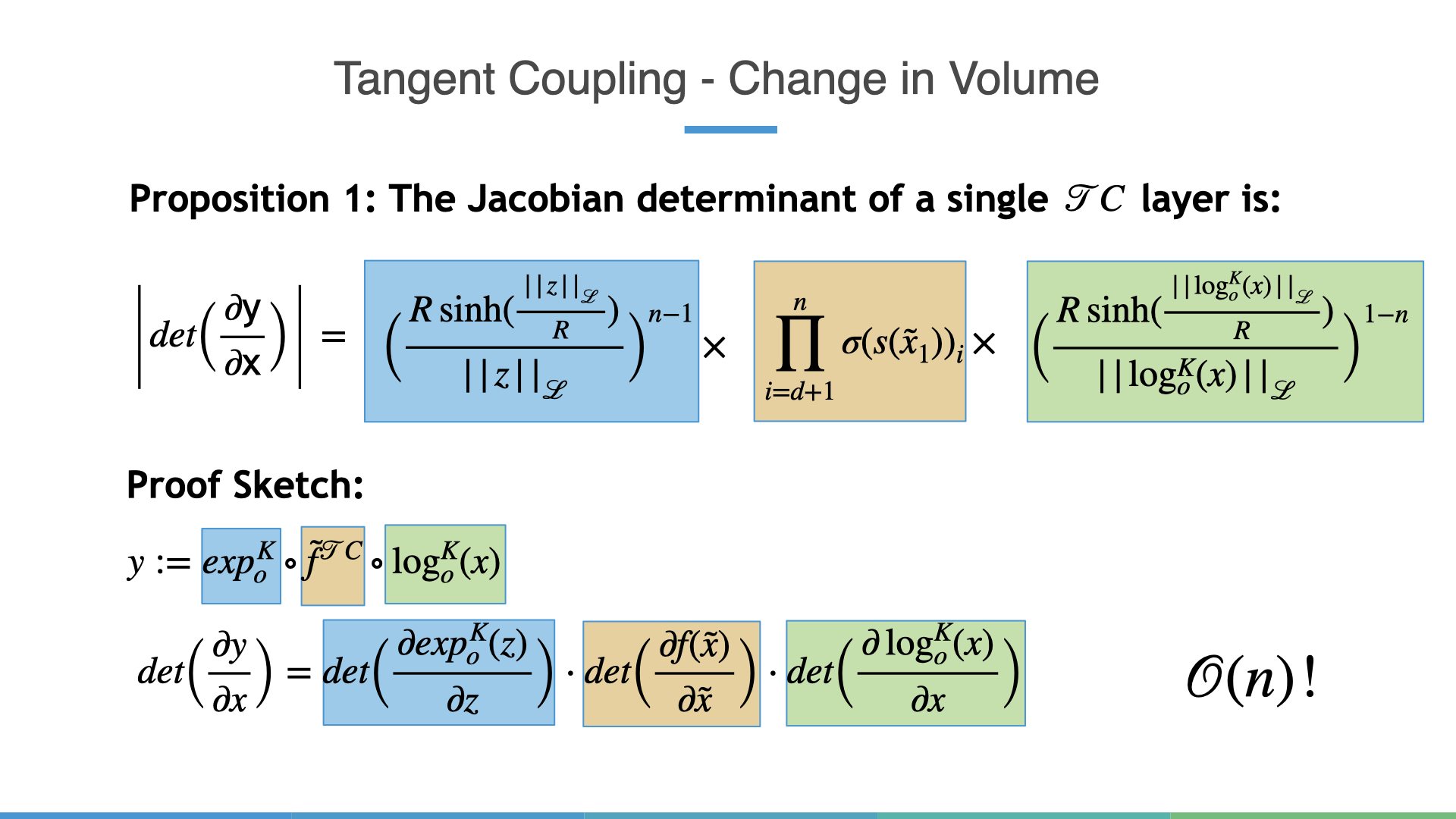The proof for this requires is a bit too heavy handed for a blog post but at a high level the only two addition terms that affect the change in volume manifest themselves through the exp and log maps (blue and green) respetively. Remarkably, the remainder of the change in volume is exactly the same as Affine Coupling!

# Normalizing Flows on Hyperbolic Spaces: Wrapped Hyperboloid Coupling

One problem that can arise with Tangent Coupling is that we only use the tangent space at the origin. This could potentially limit the expressivity of the learned distributions as we don’t explicitly use other regions of the hyperboloid. Intuitively, this implies we need to move vectors to other tangent spaces and what better way than using parallel transport! The main idea in what I call “Wrapped Hyperboloid Coupling” is that instead of simply applying a translation transformation to a set of indices in one tangent space we can use $$t$$ to predict which tangent space we want to parallel transport too! This is all fine because parallel transport is an invertible operation, much like the exponential and logarithmic maps. In equations, one $$\mathcal{W}\mathbb{H}C$$ layer is defined as:The associated change in volume, while a bit more complex than a $$\mathcal{T}C$$ layer happens to be still $$\mathcal{O}(n)$$. The proof however is a lot more involved and I’ll guide the interested reader back to the Appendix of the paper. In pictures however, the overall transformation is as follows: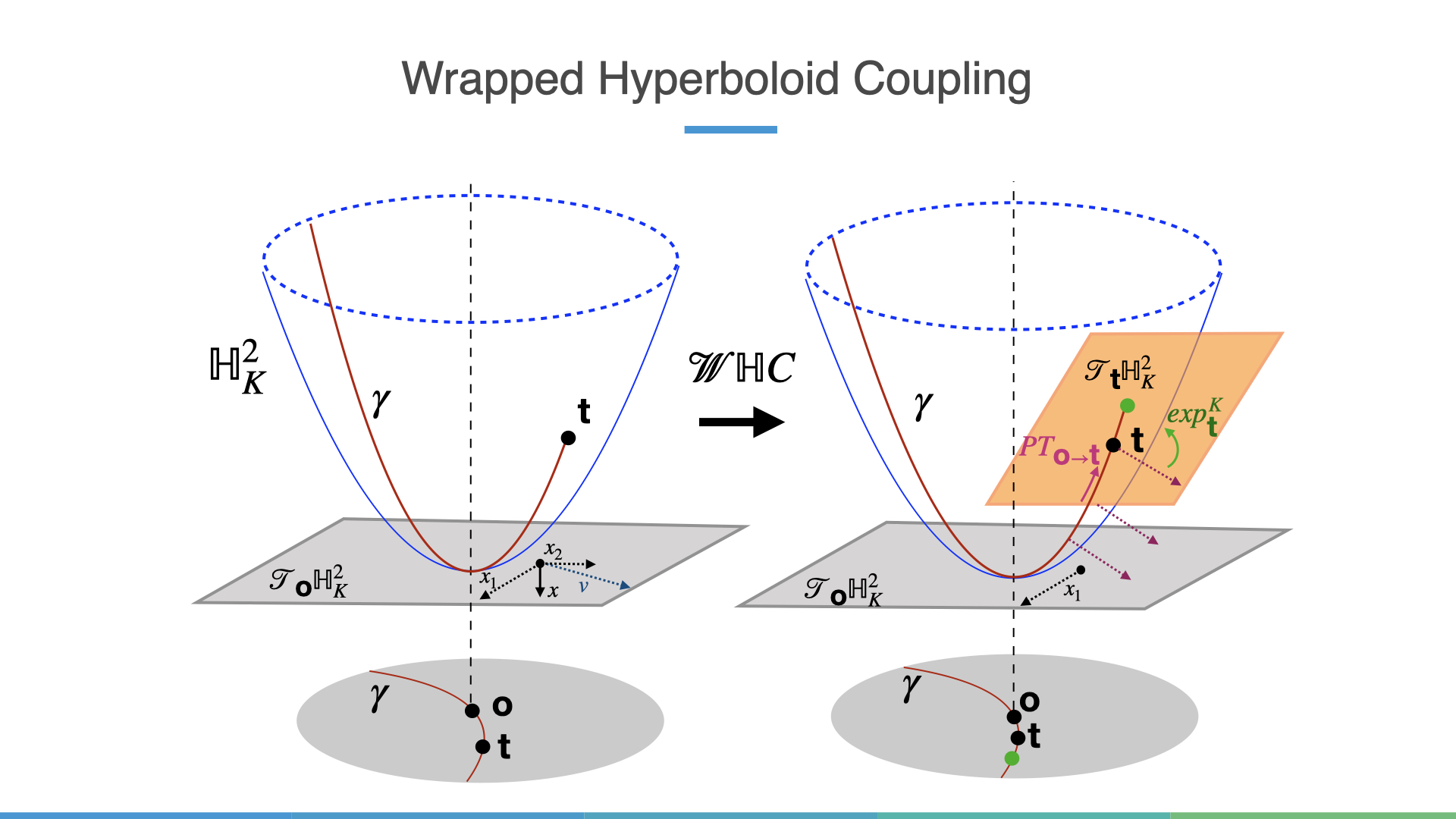Here, we move the second set of indices to the orange tangent space before using an exp map (green point).

# Go with the Flow: Experiments and Results

In this blog I’ll focus more on the qualitative results but there are also a bunch of quantitative results in the main paper. Also the code is available here so anyone reading this should feel free to build even more powerful normalizing flows!

## Density Estimation

How well does it do? Below are some visualizations of synthetic target densities which are learned by the $$\mathcal{W}\mathbb{H}C$$ Flow. $$\mathbb{H}^n_k$$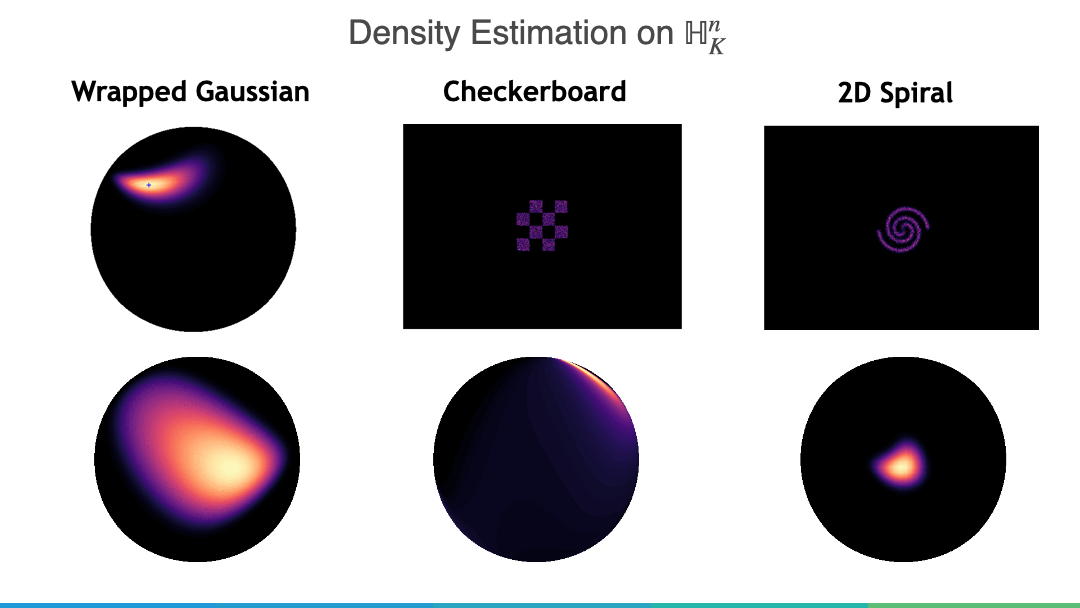We can see that the flow does almost a perfect job of learning the Wrapped Gaussian, and a reasonable job at the Checkerboard and Spiral densities. In particular multiple closeby is difficult to model but this is a known problem of Affine Coupling based flows so it’s unsurprising that similar trends hold in hyperbolic space.

## Graph Generation

We can also generate random trees and lobster graphs (a specific type of tree with a long spine). The generative model is a simple extension of a VAE to the graph setting VGAE and the Normalizing Flow is used to learn a more flexible posterior.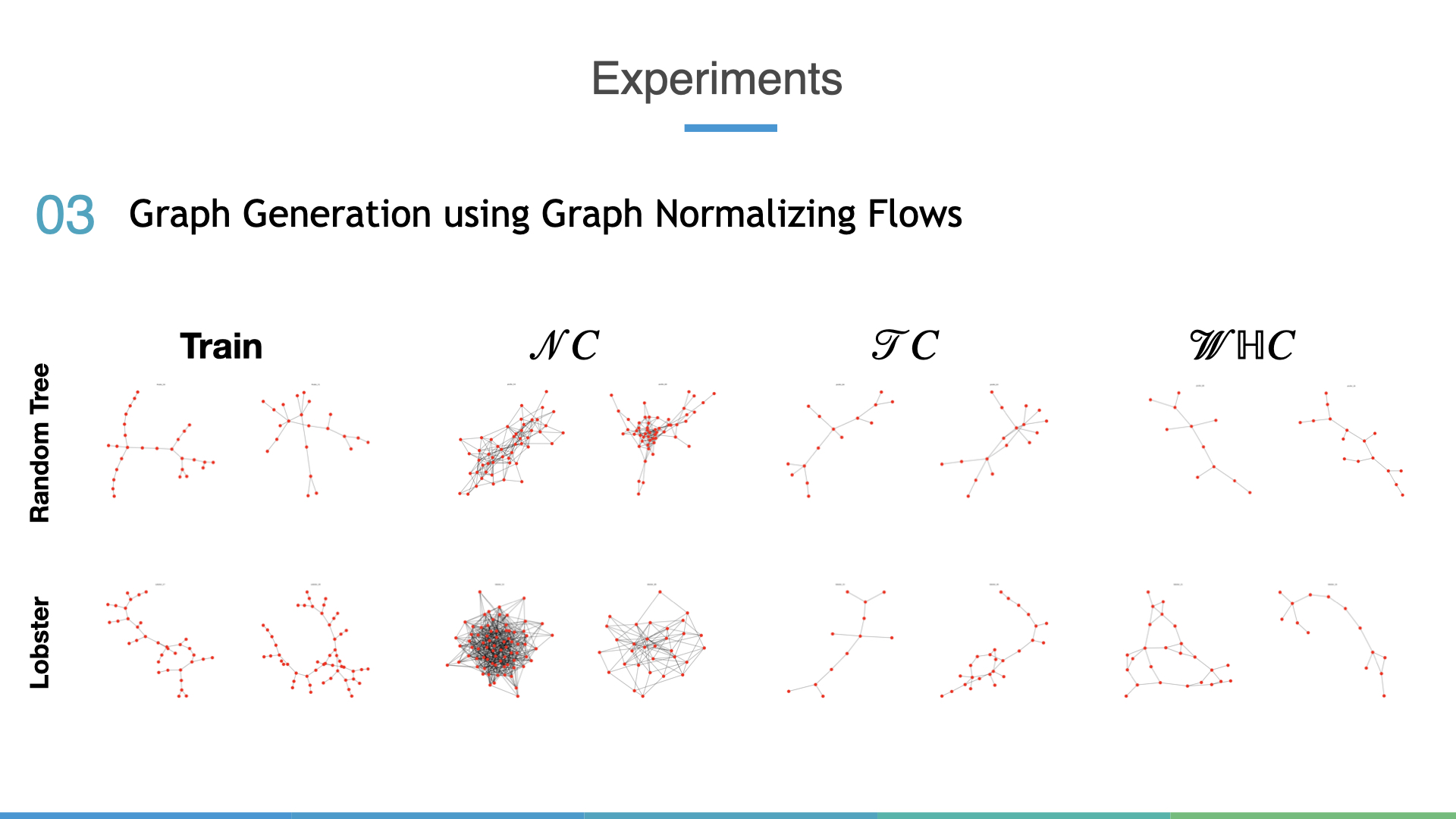Interestingly, the conventional Euclidean Normalizing Flow which happens to be an instance of Graph Normalizing Flow but still fails to capture the hierarchical structure in generated samples. Both hyperbolic flows do a much better job at learning the actual data distribution.

# Conclusion

In conclusion, hyperbolic spaces offer a great way to represent hierarchical data and the paper presented in this blog is the first step towards building one breed of generative models in Normalizing Flows. There are a bunch of super exciting future directions that I hope to try out in the future, such building non-Coupling based flows, general flows for product spaces, and the great open challenge of flows for general Riemannian manifolds.